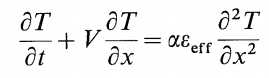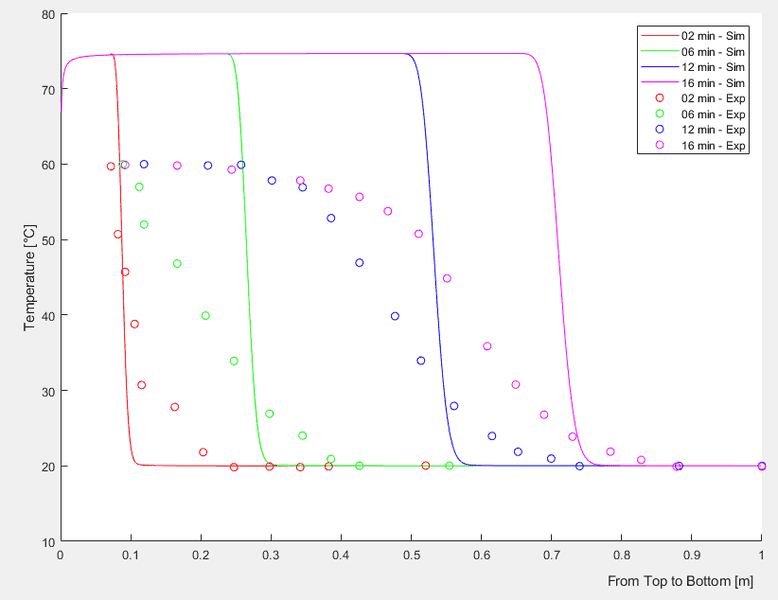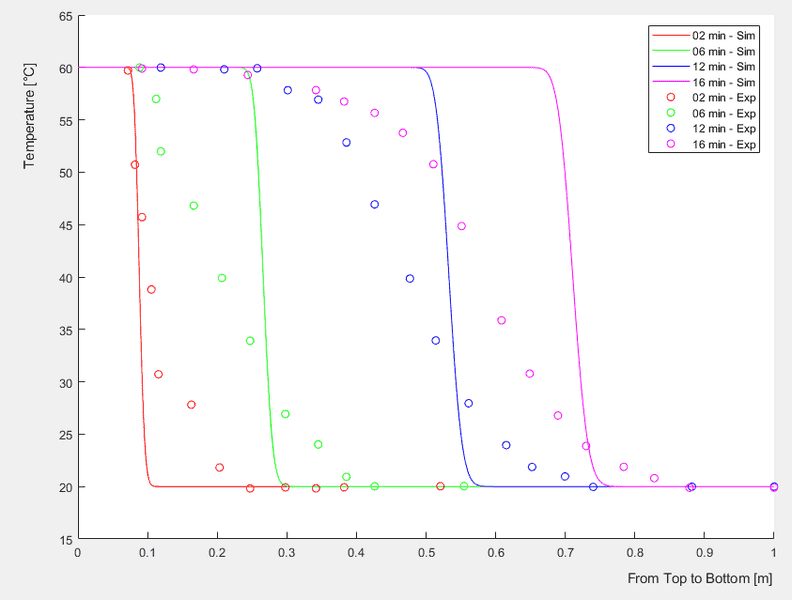# 1D Convection Diffusion Equation - Inlet Mixing Effect

• A
• HumanistEngineer
In summary, the conversation is about solving a 1D convection-diffusion equation using a Matlab code to model a sensible stratified storage tank. The issue being discussed is the abnormal temperature increase that occurs when including an additional mixing effect in the simulation. The person is seeking help in understanding why this problem is occurring and if there is a solution to it. The conversation also includes some details about the code and the parameters used in the simulation.f

#### HumanistEngineer

I have a working Matlab code solving the 1D convection-diffusion equation to model sensible stratified storage tank by use of Crank-Nicolson scheme (without εeff in the below equation).As indicated by Zurigat et al; there is an additional mixing effect having a hyperbolic decaying form from the top of the tank to the bottom (at the inlet we have a high rate of mixing). So I try to involve εeff as a magnifying effect on the thermal diffusivity as shown in the equation above. Please see the section Inlet Mixing in the Matlab code below.

The problem is that: there occurs an abnormal temperature increase more than the coming inlet temperature whenever I involve the mixing effect as a hyperbolic decaying form (please see pictures below).

Would you please tell me why I have this abnormal temperature increase problem? I tried also Forward-Time Central Space method and CN with upwind treatment but at all approaches, I encountered this abnormal issue. Any solution please? I am not so experienced with the finite difference methods!

With εeff involved, the problem with abnormal temperature increase encountered:The case without εeff, the solution of convection-diffusion equation for a stratified sensible storage tank by use of Crank-Nicolson scheme:Matlab Code:

Code:
function [T,dh,dt] = CDR_CrankNicolson_Exp_InletMixing(t_final,n)
%% 1D Convection-Diffusion-Reaction (CDR) equation - Crank-Nicolson scheme
%   solves a d2u/dx2 + b du/dx + c u = du/dt

%% References
% pg. 28 - http://www.ehu.eus/aitor/irakas/fin/apuntes/pde.pdf
% pg. 03 - https://www.sfu.ca/~rjones/bus864/notes/notes2.pdf
% http://nptel.ac.in/courses/111107063/24

tStart = tic; % Measuring computational time

%% INPUT
% t_final   : overall simulation time [s]
% n         : Node number [-]

%% Tank Dimensions
h_tank=1;       % [m]       Tank height
d_tank=0.58;     % [m]       Tank diameter
vFR=11.75;        % [l/min]   Volumetric Flow Rate
v=((vFR*0.001)/60)/((pi*d_tank^2)/4);    % [m/s]     Water velocity

dh=h_tank/n;       % [m] mesh size

dt=1;             % [s] time step
maxk=round(t_final/dt,0);    % Number of time steps

% Constant CDR coefficients | a d2u/dx2 + b du/dx + c u = du/dt
a_const=0.15e-6;     % [m2/s]Thermal diffusivity
b=-v;                        % Water velocity
c=0;                         %! Coefficient for heat loss calculation

%% Inlet Mixing - Decreasing hyperbolic function through tank height
eps_in=2;             % Inlet mixing magnification on diffusion
A_hyp=(eps_in-1)/(1-1/n);
B_hyp=eps_in-A_hyp;

Nsl=1:1:n;

eps_eff=A_hyp./Nsl+B_hyp;

a=a_const.*eps_eff;         % Case 0
% a=ones(n,1)*a_const;      % Case 1
% a=ones(n,1)*a_const*20;    % Case 2

%% Initial condition - Tank water at 20 degC
T=zeros(n,maxk);

T(:,1)=20;

%% Boundary Condition - Inlet temperature at 60 degC
T(1,:)=60;

%% Formation of Tridiagonal Matrices
%   Tridiagonal Matrix @Left-hand side
subL(1:n-1)=-(2*dt*a(1:n-1)-dt*dh*b);             % Sub diagonal - Coefficient of u_i-1,n+1
maiL(1:n-0)=4*dh^2+4*dt*a-2*dh^2*dt*c;          % Main diagonal - Coefficient of u_i,n+1
supL(1:n-1)=-(2*dt*a(1:n-1)+dt*dh*b);             % Super diagonal - Coefficient of u_i+1,n+1
tdmL=diag(maiL,0)+diag(subL,-1)+diag(supL,1);
%   Tridiagonal Matrix @Right-hand side
subR(1:n-1)=2*dt*a(1:n-1)-dt*dh*b;                % Sub diagonal - Coefficient of u_i-1,n
maiR(1:n-0)=4*dh^2-4*dt*a-2*dh^2*dt*c;          % Main diagonal - Coefficient of u_i,n
supR(1:n-1)=2*dt*a(1:n-1)+dt*dh*b;               % Super diagonal - Coefficient of u_i+1,n
tdmR=diag(maiR,0)+diag(subR,-1)+diag(supR,1);

%% Boundary Condition - Matrices
tdmL(1,1)=1; tdmL(1,2)=0;
tdmL(end,end-1)=0; tdmL(end,end)=1;

tdmR(1,1)=1; tdmR(1,2)=0;
tdmR(end,end-1)=1; tdmR(end,end)=0;

MMtr=tdmL\tdmR;

%% Solution - System of Equations
for j=2:maxk % Time Loop
Tpre=T(:,j-1);

T(:,j)=MMtr*Tpre;

if T(end,j)>=89.9
T(:,j+1:end)=[];
finishedat=j*dt;
ChargingTime=sprintf('Charging time is %f [s]', finishedat)
tElapsed = toc(tStart);
SimulationTime=sprintf('Simulation time is %f [s]',tElapsed)
return
end
end

end

#### Attachments

It's not clear what you did. What is the difference between the solid curves and the dotted curves?

Sorry Chestermiller, my mistake. The dotted curves are from an experiment while solid lines are from the simulation (the Matlab code given). So the aim of the simulation is to obtain the temperature distribution through the tank height at one dimension by solving the convection-diffusion equation dT/dt = -v dT/dx + alpha d2T/dx2. I could obtain my solution when Crank-Nicolson scheme is applied if eps_eff (inlet mixing effect) not included but far different than the experimental data (please see the second graph). After applying eps_eff as an hyperbolic decaying form as from the top of the tank to the bottom, there occurs an abnormal temperature increase in the solution (please see the first graph). I try to understand/get rid off these abnormal temperature increase so asking you.

I don't want to slog through your code to try to find an error. I'm a little confused about your spatial finite difference scheme. First you mention central differencing, and then you mention upwind differencing. Are you saying that you use central differencing on the diffusion term and upwind differencing on the advection term?

I don't want to slog through your code to try to find an error. I'm a little confused about your spatial finite difference scheme. First you mention central differencing, and then you mention upwind differencing. Are you saying that you use central differencing on the diffusion term and upwind differencing on the advection term?

For the graphs given, I used the Crank-Nicolson scheme (the graphs given are from that). However, I also mentioned that in other attempts (results not given here) I also tried different schemes (i.e. Forward time central space, Crank-Nicolson with Upwind Treatment (the hybrid scheme with the Upwind scheme on the convection/advection term), and Backward Euler) and encountered the same problem. So at each of these difference schemes applied, the solution was obtained with abnormal temperature increase (at each without the involvement of εeff, the results, the temperature distribution through the tank height is reasonable).

My recent discovery on this issue is that: when the 'effective diffusivity factor - εeff ' has a hyperbolic decaying form (high at the top unity at the bottom) this issue with abnormal temperature increase occurs (the first graph). This is because I just give a try of multiplying a constant εeff with the thermal diffusivity α. Even at high values of constant εeff there was no abnormal temperature increase obtained but a high diffusion through the tank height, as physically expected.

For the graphs given, I used the Crank-Nicolson scheme (the graphs given are from that). However, I also mentioned that in other attempts (results not given here) I also tried different schemes (i.e. Forward time central space, Crank-Nicolson with Upwind Treatment (the hybrid scheme with the Upwind scheme on the convection/advection term), and Backward Euler) and encountered the same problem. So at each of these difference schemes applied, the solution was obtained with abnormal temperature increase (at each without the involvement of εeff, the results, the temperature distribution through the tank height is reasonable).

My recent discovery on this issue is that: when the 'effective diffusivity factor - εeff ' has a hyperbolic decaying form (high at the top unity at the bottom) this issue with abnormal temperature increase occurs (the first graph). This is because I just give a try of multiplying a constant εeff with the thermal diffusivity α. Even at high values of constant εeff there was no abnormal temperature increase obtained but a high diffusion through the tank height, as physically expected.
Are you saying that you are making ##\epsilon## vary spatially from top to bottom? If so, shouldn't the diffusion term be ##\frac{\partial}{\partial x}\left(\alpha \epsilon\frac{\partial T}{\partial x}\right)##?

If I take your equation and add ##\frac{\partial (\alpha \epsilon)}{dx}\frac{\partial T}{\partial x}## to both sides, I get: $$\frac{\partial T}{\partial t}+\left[V+\frac{\partial (\alpha \epsilon)}{dx}\right]\frac{dT}{dx}=\frac{\partial}{\partial x}\left(\alpha \epsilon\frac{\partial T}{\partial x}\right)$$

•HumanistEngineer
Are you saying that you are making ##\epsilon## vary spatially from top to bottom? If so, shouldn't the diffusion term be ##\frac{\partial}{\partial x}\left(\alpha \epsilon\frac{\partial T}{\partial x}\right)##?

If I take your equation and add ##\frac{\partial (\alpha \epsilon)}{dx}\frac{\partial T}{\partial x}## to both sides, I get: $$\frac{\partial T}{\partial t}+\left[V+\frac{\partial (\alpha \epsilon)}{dx}\right]\frac{dT}{dx}=\frac{\partial}{\partial x}\left(\alpha \epsilon\frac{\partial T}{\partial x}\right)$$

That's right. I shall update the solution for the diffusion term. Thanks a lot, Chestermiller.

There is a much better way of setting up the difference equation for this problem to get much better accuracy, by eliminating numerical dispersion in the solution. In an advection-diffusion problem like this, numerical dispersion (false dispersion due to the numerical scheme) is always an issue.

The CN version of this scheme is as follows:
$$\frac{T(x+\Delta x, t+\Delta t)-T(t,x)}{\Delta t}=\frac{1}{2}\left[\frac{\partial }{\partial x}\left(\alpha \epsilon\frac{\partial T}{\partial x}\right)\right]_{(x-\Delta x),t}+\frac{1}{2}\left[\frac{\partial }{\partial x}\left(\alpha \epsilon\frac{\partial T}{\partial x}\right)\right]_{x,(t+\Delta t)}$$with, specifically, ##\Delta t=\Delta x/V##